SSC  >  Test: Fluid Mechanics- 1

# Test: Fluid Mechanics- 1

Test Description

## 20 Questions MCQ Test Mock Test Series of SSC JE Civil Engineering | Test: Fluid Mechanics- 1

Test: Fluid Mechanics- 1 for SSC 2023 is part of Mock Test Series of SSC JE Civil Engineering preparation. The Test: Fluid Mechanics- 1 questions and answers have been prepared according to the SSC exam syllabus.The Test: Fluid Mechanics- 1 MCQs are made for SSC 2023 Exam. Find important definitions, questions, notes, meanings, examples, exercises, MCQs and online tests for Test: Fluid Mechanics- 1 below.
Solutions of Test: Fluid Mechanics- 1 questions in English are available as part of our Mock Test Series of SSC JE Civil Engineering for SSC & Test: Fluid Mechanics- 1 solutions in Hindi for Mock Test Series of SSC JE Civil Engineering course. Download more important topics, notes, lectures and mock test series for SSC Exam by signing up for free. Attempt Test: Fluid Mechanics- 1 | 20 questions in 12 minutes | Mock test for SSC preparation | Free important questions MCQ to study Mock Test Series of SSC JE Civil Engineering for SSC Exam | Download free PDF with solutions
 1 Crore+ students have signed up on EduRev. Have you?
Test: Fluid Mechanics- 1 - Question 1

### For Bernoulli’s equation to remain valid, which of the following is NOT required?

Detailed Solution for Test: Fluid Mechanics- 1 - Question 1

The conditions to be satisfied for the applicability of Bernoulli’s equation are

I. Flow along a stream line

II. Flow is steady and Incompressible

III. Effect of viscous forces is negligible

Test: Fluid Mechanics- 1 - Question 2

### The hydraulic grade line for flow in a pipe of constant internal diameter ______.

Detailed Solution for Test: Fluid Mechanics- 1 - Question 2

For the pipe of constant diameter, the hydraulic gradient line is always sloping down in the direction of flow and is parallel to total energy line and the difference between both the lines represents velocity head. Due to hf(head loss due to friction) along the length of pipe, H.G.L always sloping down.

Test: Fluid Mechanics- 1 - Question 3

### A hydraulic jump occurs when the grade changes from:

Detailed Solution for Test: Fluid Mechanics- 1 - Question 3

When the flow conditions changes form super critical (Fr > 1) to subcritical (Fr < 1), this results to an abrupt rise of water accompanied by turbulent rollers is called as hydraulic Jump or standing wave.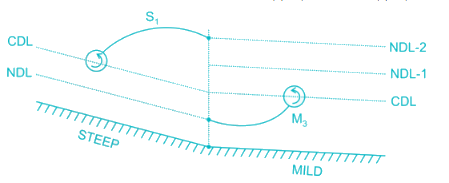The hydraulic Jump occurs when the grade changes from sleep to mild

Test: Fluid Mechanics- 1 - Question 4

In an orifice used for flow measurement, the coefficient of velocity is always:

Detailed Solution for Test: Fluid Mechanics- 1 - Question 4

Coefficient of velocity through orifice =ActualvelocityTheoreticalvelocity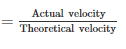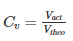Vtheo > Vact

So, Cv is always less than 1.

Test: Fluid Mechanics- 1 - Question 5

The centre of pressure on inclined immersed surface remains valid for the centre of pressure on vertical immersed surface, if the angle (θ) at immersed surface with liquid surface is:

Detailed Solution for Test: Fluid Mechanics- 1 - Question 5

Centre of Pressure on a Immersed surface from water surface is given by: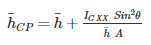For vertical surface θ = 90°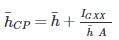So, the formula is valid for θ = 90°

Test: Fluid Mechanics- 1 - Question 6

The mechanical efficiency of an impulse turbine is generally between:

Detailed Solution for Test: Fluid Mechanics- 1 - Question 6

Mechanical efficiency of Impulse turbine is given by: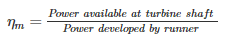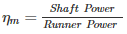It generally varies between 97 to 99

Also, volumetric efficiency (ηv) = 97 to 99

Overall efficiency (ηo) = 85 to 90

Test: Fluid Mechanics- 1 - Question 7

Which of the following is CORRECT about the viscosity of gas?

Detailed Solution for Test: Fluid Mechanics- 1 - Question 7

As temperature increases, randomness in molecules also increases. This increase in randomness will result in increase in viscosity as viscosity of gas depends on randomness & collision of gas molecules.

Phenomenon: As a gas is heated, the movement of gas molecules increases and the probability that one gas molecule will collide with another gas molecule increases. In other words, increasing gas temperature causes the gas molecules to collide more often. This increases the gas viscosity because the transfer of momentum between stationary and moving molecules is what causes gas viscosity.

Test: Fluid Mechanics- 1 - Question 8

Pressure of 200 kPa is equivalent to the head of z metre of liquid having relative density 1.59. The value of z (m) is _____.

Detailed Solution for Test: Fluid Mechanics- 1 - Question 8

200 kPa = 200,000 Pa

200,000 Pa = 200,000 N/m²

Pressure in liquids is calculate by the formula:

P = αgh

Where P = pressure, α = density, g = force of gravity,  and h = height

Taking g = 10

αgh = 200,000

1590 x 10 x h = 200,000

h = 200,000/15900

h = 12.58 m

Test: Fluid Mechanics- 1 - Question 9

A longitudinal rectangular surface is hanged into the water such that its top and bottom points are at depth of 1.5 m and 6.0 m respectively. The depth of center of pressure (m) from the top surface is _____.

Detailed Solution for Test: Fluid Mechanics- 1 - Question 9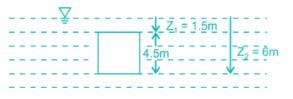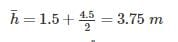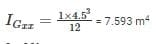θ = 90°

A = 1 × 4.5 m2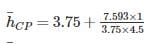h¯CP= 4.2 metre

Test: Fluid Mechanics- 1 - Question 10

The velocity potential which follow the equation of continuity is ________

Detailed Solution for Test: Fluid Mechanics- 1 - Question 10

Velocity Potential function (ϕ) is given as = x2 - y2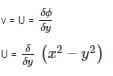u = - 2xV = 2y

For continuity to be satisfied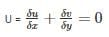-2 + 2 = 0

(Hence satisfied)

Test: Fluid Mechanics- 1 - Question 11

The term alternate depths in open channel flow is used to designate the depths

Detailed Solution for Test: Fluid Mechanics- 1 - Question 11

For a given discharge Q in a channel, there will be two depths for a given specific energy. These two depths are known as alternate depths.

Test: Fluid Mechanics- 1 - Question 12

If a thin plate is held parallel to fluid stream the pressure drag on it is:

Detailed Solution for Test: Fluid Mechanics- 1 - Question 12

Viscous forces and pressure forces are responsible for drag forces. There are two types of drag forces present pressure drag and skin friction drag. Pressure drag is due to the wake region behind the body and skin friction drag is due to the viscosity of the fluid.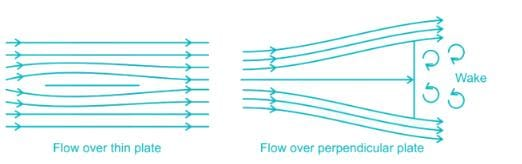In case of flow over a thin plate placed parallel to a stream of flowing fluid, the flow separation (if occurs), would occur only towards the rear end and the wake is negligibly small. The resulting pressure drag will also be very small, approximate to zero.

On the other hand, pressure drag will be large when the thin plate is held perpendicular to the flow.

Test: Fluid Mechanics- 1 - Question 13

Two pumps can operate independently at heads H1, H2 and discharges Q1, Q2, respectively. If the pumps are connected in parallel, then what are the resulting discharge (Q) and head (H)?

Detailed Solution for Test: Fluid Mechanics- 1 - Question 13

Pumps in Parallel:

Same Head: H = H1 = H2

Add the discharge: Q = Q1 + Q2

Pumps in Series:

Same Discharge: Q = Q1 = Q2

Test: Fluid Mechanics- 1 - Question 14

An isentropic process is always...

Detailed Solution for Test: Fluid Mechanics- 1 - Question 14

In thermodynamics an isentropic process is an idealized thermodynamic process. that is both adiabatic and reversible. The work transfer of system is friction-less and there is no transfer of heat as matter.

Test: Fluid Mechanics- 1 - Question 15

A centrifugal pump delivers a liquid when pressure rise in impeller is equal to _______

Detailed Solution for Test: Fluid Mechanics- 1 - Question 15

Manometric head is the head against which head is required to be produced by the pump to deliver water to the destination. Manometric head is higher than the sum of suction and delivery heads because it accounts for head losses due to friction.

Test: Fluid Mechanics- 1 - Question 16

Separation of flow occurs when pressure gradient

Detailed Solution for Test: Fluid Mechanics- 1 - Question 16

Flow separation occurs when the pressure gradient is positive and velocity gradient is negative.

Test: Fluid Mechanics- 1 - Question 17

The most economical section of circular channel for maximum discharge is obtained when (Where, d is the diameter of circular section)

Detailed Solution for Test: Fluid Mechanics- 1 - Question 17

D = depth of flow

d = diameter of pipe

Rm = Hydraulic Mean depth = A/P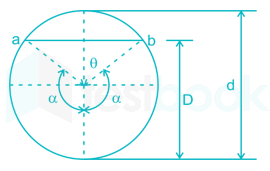Perimeter, P = αd

Condition for Maximum Discharge for Circular Section:

α = 154° = 2.65 radians

D = 0.95 d

Rm = 0.29 d

Condition for Maximum Velocity for Circular Section:

α = 128.75° = 2.25 radians

D = 0.81 d

Rm = 0.3 d

Test: Fluid Mechanics- 1 - Question 18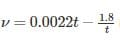is the equation to determine kinematic viscosity of liquids by

Detailed Solution for Test: Fluid Mechanics- 1 - Question 18

The device used for measurement of viscosity is known as viscometer. According to Saybolt viscometer: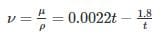Test: Fluid Mechanics- 1 - Question 19

A pump delivers water at a rate of 0.025 m3/s against a head of 30 m. If the overall efficiency of the pump is 75%, the power required by the pump is:

Detailed Solution for Test: Fluid Mechanics- 1 - Question 19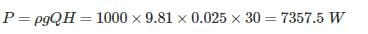Power required: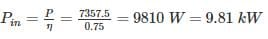Test: Fluid Mechanics- 1 - Question 20

A streamlined body is defined as a body about which ________.

Detailed Solution for Test: Fluid Mechanics- 1 - Question 20

A stream lined body is defined as the body whose surface coincides with the stream - lines, when the body is placed in a flow. In that case the separation of flow will take place only at trailing edge (or rearmost part of the body).

Behind a stream lined body, wake formation zone will be very small and consequently the pressure drag will be very small. Thus the total drag on the stream lined body will be due to friction (shear) only.

## Mock Test Series of SSC JE Civil Engineering

1 videos|1 docs|64 tests
 Use Code STAYHOME200 and get INR 200 additional OFF Use Coupon Code
Information about Test: Fluid Mechanics- 1 Page
In this test you can find the Exam questions for Test: Fluid Mechanics- 1 solved & explained in the simplest way possible. Besides giving Questions and answers for Test: Fluid Mechanics- 1, EduRev gives you an ample number of Online tests for practice

## Mock Test Series of SSC JE Civil Engineering

1 videos|1 docs|64 tests